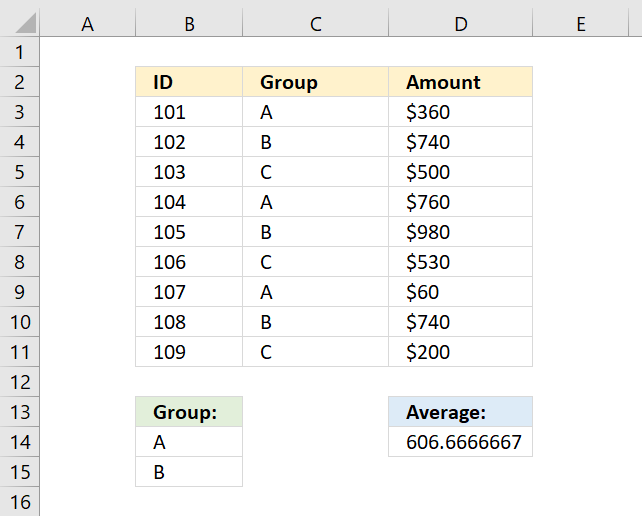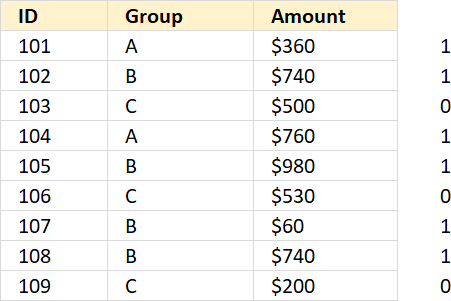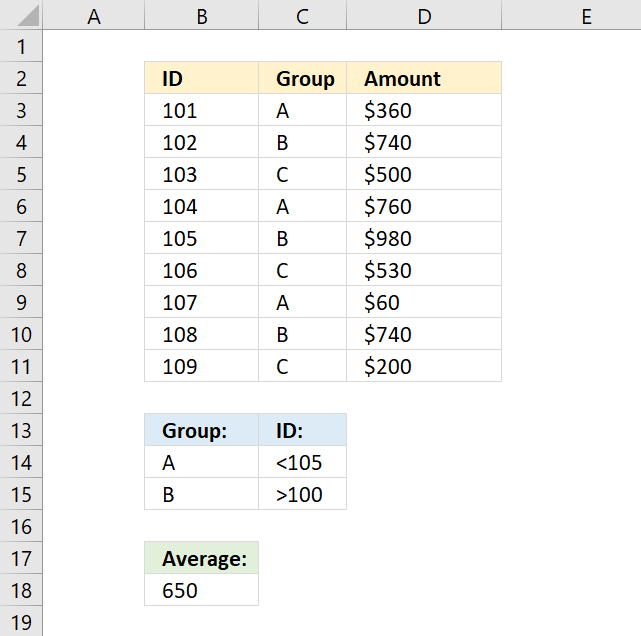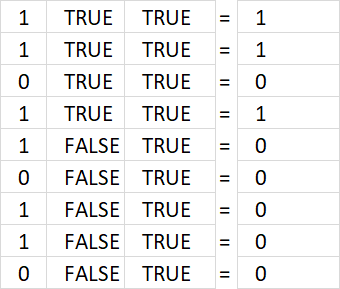Author: Oscar Cronquist Article last updated on January 03, 2022This article demonstrates two formulas that calculate averages, the first formula calculates an average based on criteria, and the second formula uses both criteria and two other conditions applied to an adjacent column using AND logic.

## 1. AVERAGE based on criteriaThe array formula in cell D14 calculates an average based on multiple criteria in cell range B14:B15. If value in column C is equal to B14 or B15 the amount in column D on the same row is included in the average.

=AVERAGE(IF(COUNTIF(B14:B15,C3:C11),D3:D11,""))

### 1.1 How to enter an array formula

To enter an array formula, type the formula in a cell then press and hold CTRL + SHIFT simultaneously, now press Enter once. Release all keys.

The formula bar now shows the formula with a beginning and ending curly bracket telling you that you entered the formula successfully. Don't enter the curly brackets yourself.

### 1.2 Explaining formula in cell D14

#### Step 1 - Count cells based on criteria

You can't use AVERAGEIF or AVERAGEIFS function in this example, as far as I know. The COUNTIF function allows you to check if at least one out of multiple values are found in a cell range.

Note that I am using multiple values in the second argument, the COUNTIF function returns an array that indicates where group A or B is.

COUNTIF(rangecriteria)

COUNTIF(B14:B15, C3:C11)

becomes

COUNTIF({"A";"B"},{"A";"B";"C";"A";"B";"C";"B";"B";"C"})

and returns {1; 1; 0; 1; 1; 0; 1; 1; 0}. The image below shows the array next to column Amount.If group is "A" or "B" the corresponding cell on the same row is 1 in the column without a name (next to column Amount).

#### Step 2 - Replace 1 with the corresponding amount

The IF function lets you filter values in column D based on the COUNTIF function that serves as a logical test in this case.

IF(logical_test, [value_if_true], [value_if_false])

IF(COUNTIF(B14:B15, C3:C11), D3:D11, "")

becomes

IF({1; 1; 0; 1; 1; 0; 1; 1; 0}, D3:D11, "")

and returns

{360; 740; ""; 760; 980; ""; 60; 740; ""}

#### Step 3 - Calculate average

The AVERAGE function then returns the average from the values in the array ignoring the blanks.

AVERAGE(number1[number2], ...)

AVERAGE(IF(COUNTIF(B14:B15,C3:C11),D3:D11,""))

becomes

AVERAGE({360;740;"";760;980;"";60;740;""})

returns 606.6667 in cell D14.

## 2. AVERAGE - AND OR logicThe following array formula calculates an average based on two conditions, if group is equal to A OR B AND ID is less than 105 AND larger than 100.

=AVERAGE(IF(COUNTIF(B14:B15, C3:C11)*(B3:B11<105)*(B3:B11>100), D3:D11,""))

How to enter an array formula

### 2.1 Explaining formula

#### Step 1 - First condition

The COUNTIF function allows you to check if at least one out of multiple values are found in a cell range.

Note that I am using multiple values in the second argument, the COUNTIF function returns an array that indicates where group A or B is.

COUNTIF(rangecriteria)

COUNTIF(B14:B15, C3:C11)

becomes

COUNTIF({"A"; "B"},{"A"; "B"; "C"; "A"; "B"; "C"; "A"; "B"; "C"})

and returns {1; 1; 0; 1; 1; 0; 1; 1; 0}.

#### Step 2 - Second condition

The less than character is a logical operator that checks if the numbers in B3:B11 are smaller than 105, the result is a boolean value TRUE or FALSE.

B3:B11<105

becomes

{101; 102; 103; 104; 105; 106; 107; 108; 109}<105

and returns

{TRUE; TRUE; TRUE; TRUE; FALSE; FALSE; FALSE; FALSE; FALSE}.

#### Step 3 - Third condition

The greater than character is a logical operator that checks if the numbers in B3:B11 are larger than 100.

B3:B11>100

becomes

{101; 102; 103; 104; 105; 106; 107; 108; 109}>100

and returns

{TRUE; TRUE; TRUE; TRUE; TRUE; TRUE; TRUE; TRUE; TRUE}.

#### Step 4 - Multiply arrays

There are three logical expressions in the first IF argument COUNTIF(B14:B15,C3:C11)*(B3:B11<105)*(B3:B11>100).

The asterisk multiplies the arrays, 1 indicates a location where all three logical tests return TRUE and FALSE if at least one returns FALSE.The array returned from the COUNTIF function is in the first column above, B3:B11<105 is in the second column, and B3:B11>100 is in the third column.

The first row: 1*TRUE*TRUE equals 1. All three logical tests are TRUE.

COUNTIF(B14:B15, C3:C11)*(B3:B11<105)*(B3:B11>100)

becomes

{1; 1; 0; 1; 1; 0; 1; 1; 0}*{TRUE; TRUE; TRUE; TRUE; FALSE; FALSE; FALSE; FALSE; FALSE}*{TRUE; TRUE; TRUE; TRUE; TRUE; TRUE; TRUE; TRUE; TRUE}

and returns

{1; 1; 0; 1; 0; 0; 0; 0; 0}.

#### Step 5 - Replace 1 with the corresponding amount

The IF function lets you filter values in column D based on the COUNTIF function that serves as a logical test in this case.

IF(logical_test, [value_if_true], [value_if_false])

The corresponding value on the same row is 360 and is included in the AVERAGE calculation.

IF(COUNTIF(B14:B15, C3:C11)*(B3:B11<105)*(B3:B11>100), D3:D11,"")

becomes

IF({1; 1; 0; 1; 0; 0; 0; 0; 0}, D3:D11,"")

becomes

IF({1; 1; 0; 1; 0; 0; 0; 0; 0}, {360; 740; 500; 760; 980; 530; 60; 740; 200},"")

and returns

{360; 740; ""; 760; ""; ""; ""; ""; ""}.

#### Step 6 - Calculate average

The AVERAGE function then returns the average from the values in the array ignoring the blanks.

AVERAGE(number1[number2], ...)

AVERAGE(IF(COUNTIF(B14:B15, C3:C11)*(B3:B11<105)*(B3:B11>100), D3:D11,""))

becomes

AVERAGE({360; 740; ""; 760; ""; ""; ""; ""; ""})

There are two more values that are also in the calculation found in the second and fourth row, 740 and 760.

The average is 620 and is displayed in cell B18.